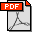N. J. Mitra, N. Gelfand, H. Pottmann, and L. Guibas. Registration of Point Cloud Data from a Geometric Optimization Perspective. Eurographics Symposium on Geometry Processing, pp. 23-32, 2004. Abstract: We propose a framework for pairwise registration of shapes represented by point cloud data (PCD). We assume that the points are sampled from a surface and formulate the problem of aligning two PCDs as a minimization of the squared distance between the underlying surfaces. Local quadratic approximants of the squared distance function are used to develop a linear system whose solution gives the best aligning rigid transform for the given pair of point clouds. The rigid transform is applied and the linear system corresponding to the new orientation is build. This process is iterated until it converges. The point-to-point and the point-to-plane Iterated Closest Point (ICP) algorithms can be treated as special cases in this framework. Our algorithm can align PCDs even when they are placed far apart, and is experimentally found to be more stable than point-to-plane ICP. We analyze the convergence behavior of our algorithm and of point-to-point and point-to-plane ICP under our proposed framework, and derive bounds on their rate of convergence. We compare the stability and convergence properties of our algorithm with other registration algorithms on a variety of scanned data. Bibtex: ```@INPROCEEDINGS{mgpg-rpcdgop-04, AUTHOR = "N.~J.~Mitra and N.~Gelfand and H.~Pottmann and L.~Guibas", TITLE = "Registration of Point Cloud Data from a Geometric Optimization Perspective", BOOKTITLE = "Symposium on Geometry Processing", YEAR = "2004", PAGES = "23--31", }```